Summary Article: VI.19 Leonhard Euler
From The Princeton Companion to Mathematics

Euler was one of the most influential and prolific mathematicians in history. His first publication was a 1726 paper on mechanics, and his last was a collection published in 1862, seventy-nine years after his death. There are over eight hundred papers bearing his name, about three hundred of them appearing posthumously, and more than twenty books. His Opera Omnia fill over eighty volumes.

In number theory, Euler introduced the Euler phi function, φ(n), to denote the number of positive integers less than n and relatively prime to n, and proved the fermat–euler theorem [III.58] that n divides aφ(n) − 1. He showed that the remainders relatively prime to n form what we now call a group under multiplication and he expanded the theory of quadratic and higher-order residues. He proved fermat’s last theorem [V.10] for n = 3. He stated that any real polynomial of degree n is a product of real and quadratic factors and has n complex roots, but was unable to give complete proofs. He was the first to use generating functions [IV.18 §§2.4, 3] when he gave a generating function for Naudé’s partition problem: the question of how many different ways a given integer can be written as a sum of positive integers. He introduced the function σ(n), the sum of the divisors of an integer n, and used this function to increase the number of known pairs of amicable numbers (a pair m, n of numbers is called amicable if the sum of the proper divisors of m equals n, and vice versa) from 3 to over 100. He showed that any prime number of the form 4n + 1 is the sum of two rational squares. lagrange [VI.22] later improved this result to show that such numbers are the sum of two integer squares. Euler factored the fifth Fermat number, F5 = 225 + 1, thus refuting fermat’s [VI.12] conjecture that all integers of the form Fn = 22n + 1 were prime. He made extensive studies of the binary quadratic forms x2 + y2, x2 + ny2, and mx2 + ny2, and proved a form of the law of quadratic reciprocity [V.28].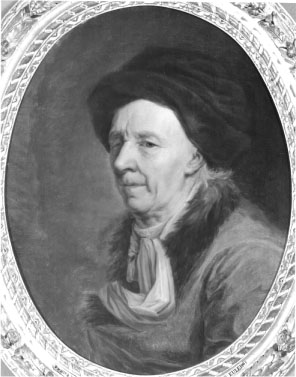Leonhard Euler

Euler was the first to use analytic methods in number theory. In the 1730s he calculated to several decimal places the so-called Euler–Mascheroni constant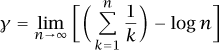and discovered many of its properties. Mascheroni added to those properties in the 1790s. Euler also discovered the sum–product formula for what we now call the Riemann zeta function,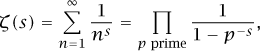and he evaluated the function for positive even values of s.

In analysis, Euler was largely responsible for shaping the modern calculus curriculum. He was also the first person to take a systematic approach to the solution of differential equations and to problems of the calculus of variations [III.94]. He discovered a differential equation sometimes called the “Euler necessary condition” and sometimes called the “Euler–Lagrange equation.” The equation tells us that if J is defined by the integral equation J = ∫ab f(x, y, y′) dx, then a function y(x) that maximizes or minimizes J will satisfy the differential equation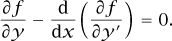Euler apparently thought that the condition was also sufficient. Very early in his career, he pioneered the use of integrating factors for solving differential equations, though the almost simultaneous published solution of Clairaut was more complete and more widely read, so credit for this innovation usually falls to Clairaut. He also did the first work using what are now called fourier series [III.27] and laplace transforms [III.91], more than a generation before laplace [VI.23] or fourier [VI.25] began doing mathematics, though they took the fields much farther than Euler had.

Much of Euler’s best work involved series. His first widely acclaimed result was when he solved one of the best-known problems of his age, the seventy-year-old “Basel problem.” The problem was to evaluate the sum of the reciprocals of the square integers, or ζ(2). Euler showed that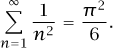(For a sketch of a proof, see π [III.70].)

He developed the Euler–Maclaurin series to strengthen the relationships between series and integrals. The existence of the Euler–Mascheroni constant followed from these researches. Using techniques he called “interpolation of series,” he developed the gamma function [III.31] and the beta function. He developed the first extensive theory of continued fractions [III.22], and derived series for the accurate and efficient calculation of logarithms [III.25 §4] and trigonometric tables, often to more than twenty decimal places.

He was the first to do calculus with complex numbers and to investigate logarithms of negative and complex numbers. This research led to a long and bitter controversy with d’alembert [VI.20].

Euler was not the first to prove that eiθ = cos θ + i sin θ or to know that eπi = −1, but he made so much more use of these facts than any of his predecessors that this last formula is generally known as Euler’s identity.

He is regarded as a pioneer in topology and graph theory for his necessary condition for a graph to have an Euler path, the so-called Königsburg bridge problem. This is to determine whether or not a graph has a path that traverses every edge exactly once. He also discovered and gave a flawed proof that, for a polyhedron “bounded by planes,” Euler’s words for what we now call “convex,” VE + F = 2, where V is the number of vertices, E is the number of edges, and F is the number of faces. (For details about the flaws in Euler’s proof, see Richeson and Francese (2006).)

Euler proved a form of the general addition theorem for elliptic integrals and gave a complete classification of elastic curves. At the command of his king, Frederick the Great of Prussia, he studied hydraulics, designed pumps and fountains, and evaluated the probabilities and combinatorics involved in the state lotteries.

In a triangle, the line on which the orthocenter, the centroid, and the circumcenter lie is the Euler line. The Euler method is an algorithm for giving numerical solutions to differential equations. The euler differential equation [III.23] is the partial differential equation that describes continuity of fluid flow.

Euler tried to use lunar and planetary theory to solve the problem of finding longitude at sea. In studying the orbit of a comet, he made the first steps in the statistics of observed data.

He left Switzerland in 1727 to work in the new academy of Peter the Great in Saint Petersburg. In 1741 he moved to Berlin and the academy of Frederick the Great, but returned to Saint Petersburg in 1766, after the ascension of Catherine the Great. He was blind for the last fifteen years of his life, during which time he nevertheless wrote over three hundred papers. He won the annual prize competition of the Paris Academy twelve times.

His series of calculus books, published in four volumes between 1755 and 1770, were the first successful calculus textbooks. It was the climax of a complete series of mathematical textbooks, including arithmetic (1738), algebra (1770), and the Introductio in Analysin Infinitorum (1748), a textbook on the mathematics Euler thought was necessary to understand calculus.

In the two volumes of the Mechanica (1736), Euler gave the first calculus-based treatment of the mechanics of point masses. He followed this with another twovolume work, Theoria Motus Corporum (1765), on the motions of solid bodies, including rotations.

Other books include Methodus Inveniendi (1744), the first unified treatment of the calculus of variations, Tentamen Novae Theoriae Musicae (1739), on the physics of music and including the first use of logarithms in the theory of pitch, three different books on celestial mechanics and lunar theory, two on the theory of shipbuilding, three on optics, and one on ballistics.

Our modern notion that functions [I.2 §2.2] are a fundamental object in mathematics is due to Euler. Euler standardized the use of the symbols e, π, and i, as well as ∑ for summations and Δ for finite differences.

His Letters to a German Princess in three volumes (1768–71) is regarded variously as the first work of popular science writing by a first-rate scientist and as an important work in the philosophy of science.

Laplace is reported to have advised, “Read Euler. Read Euler. He is the master of us all.” The words are probably not those of Laplace, but the misattribution does not affect the quality of the advice.

• , and , eds. 2007. Leonhard Euler: Life, Work and Legacy. Amsterdam: Elsevier.
• 1999. Euler: the Master of Us All. Washington, , DC: Mathematical Association of America.
• 1984. Elements of Algebra. New York: Springer. (Reprint of 1840 edition. London: Longman, Orme, and Co.).
• 1988, 1990. Introduction to Analysis of the Infinite, books I and II, translated by . New York: Springer.
• 2000. Foundations of Differential Calculus, translated by . New York: Springer.
• , and . 2007. The flaw in Euler’s proof of his polyhedral formula. American Mathematical Monthly 114(1):286-96.

•Copyright © 2010 by Princeton University Press

### Related Articles

##### Full text Article Euler , Leonhard (1707 - 1783)
The Cambridge Dictionary of Scientists

Euler was the son of a Calvinist pastor who gave him much of his early education, including mathematics. Later he studied...

##### Full text Article Euler, Leonhard
Astronomy Encyclopedia

(1707-83) Swiss mathematician who worked at the Berlin Academy and the St Petersburg Academy of Science, making significant contributions to...

##### Full text Article Euler, Leonhard (1707 - 1783)
The Macmillan Encyclopedia

Swiss mathematician, regarded as the greatest mathematician of the 18th century and the most prolific ever, writing 800 papers...

See more from Credo